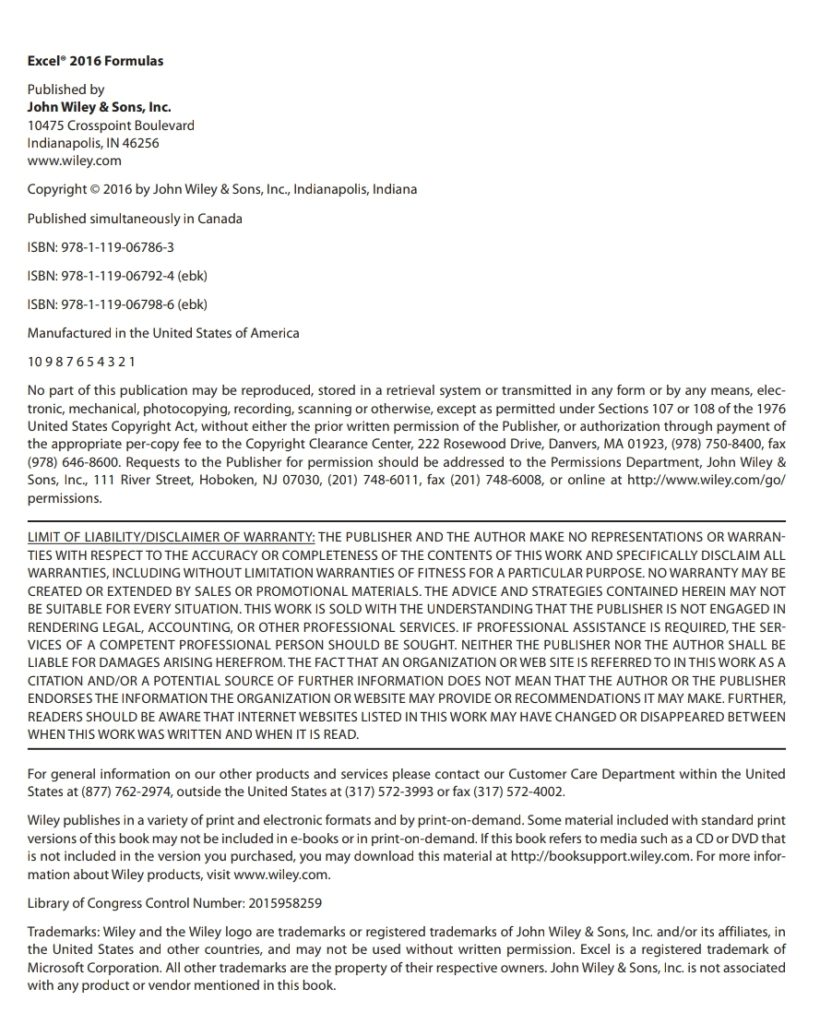Home » Download » Sách Excel 2016 Formulas – John Wiley & Sons (2016) pdf

# Sách Excel 2016 Formulas – John Wiley & Sons (2016) pdf

Pages : 818 – Year 2019 – Size 174MB – English

## DESCRIPTION

Leverage the full power of Excel formulas

Excel 2016 Formulas is fully updated to cover all of the tips, tricks, and techniques you need to maximize the power of Excel 2016 through the use of formulas. This comprehensive book explains how to create financial formulas, release the power of array formulas, develop custom worksheet functions with VBA, debug formulas, and much more. Whether you’re a beginner, a power user, or somewhere in between this is your essential go-to for the latest on Excel formulas.excel 2016 formulas inside

When conducting simple math or building highly complicated spreadsheets that require formulas up to the task, leveraging the right formula can heighten the accuracy and efficiency of your work, and can improve the speed with which you compile and analyze data. Understanding which formulas to use and knowing how to create a formula when you need to are essential.

• Access tips, tricks, and techniques that have been fully updated to reflect the latest capabilities of Microsoft Excel
• Create and use formulas that have the power to transform your Excel experience
• Leverage supplemental material online, including sample files, templates, and worksheets from the book